# Two wine

In 2:1 mix wine solution cost 4.1 USD.
In 1:2 mix wine solution cost 4.5 USD.

How much cost liter of each wine?

x =  3.7 USD/l
y =  4.9 USD/l

### Step-by-step explanation:

2x+y=3•4.1; x+2y=3•4.5

2•x+y=3•4.1
x+2•y=3•4.5

2x+y = 12.3
x+2y = 13.5

x = 37/10 = 3.7
y = 49/10 = 4.9

Our linear equations calculator calculates it.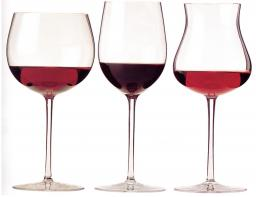Did you find an error or inaccuracy? Feel free to write us. Thank you!Tips to related online calculators
Do you have a system of equations and looking for calculator system of linear equations?

## Related math problems and questions:

• Wines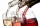Eleven liters of white wine and eight liters of red wine cost a total of 1315 kc. 1 liter of white wine was 10 kc cheaper than a liter of red wine. How much is 1 liter of white and how much red wine?
• SolutionWhat amount of 80% solution and what amount of water is needed to mix in order to obtain two liters of 60% solution of this mixture?
• SolutionsHow much 60% solution and how much 35% solution is needed to create 100 l of 40% solution?
• PharmacyAt the pharmacy are in one container 20% solution in the second 50% solution of disinfectant. They need to prepare 4 L of 48-percent solution. What amount of solution from each container is needed to mix?
• MixingIf we mix 5 kg of goods of one kind and 3 kg second one, resulting mixture cost 16.50 EUR/kg. If these quantities are mixed in reverse - first three kilograms and 5 kilograms second cost of mixture is 18.50 EUR/kg. What is the price of one kg of goods of
• Banknotes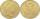$1390 was collected. How much was in$20 notes and how many in \$50 notes in that order? How many solutions exists?
• SpiritFrom 55% and 80% spirit we would like to produce 0.2 kg of 60% spirit. How many of them we must use in a solution?
• Vinegar 2How many percentages of vinegar solution will we get if we mix 3.5 liters of 5.8% and 5 liters of 7.6% vinegar?
• Hydrochloric acidDetermine the concentration of which must have a solution of hydrochloric acid that mixing 10 l of the solution with 8 liters of 26% solution to get the solution with a concentration of 50%?
• Disinfecting solutionHow much distilled water is necessary to pour into 500 ml of 33% hydrogen peroxide solution to obtain 3% disinfecting solution?
• Bottle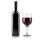Bottle with wine costs 42 USD. Wine is 11 USD more expensive than the empty bottle. How much is the bottle itself?
• Home cleaning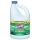Mr. Smith is cleaning up a big mess at home. In the closet, he finds a solution that is 5% bleach, and another stronger solution that is 20% bleach. For this particular job, he needs 600mL of 15% bleach. How much of each type (to the nearest mL) should he
• Mixing waterWe should fill the 30-liter container with water at 60 degrees Celsius. How many liters of water 80 degrees C hot, and how many liters of water 20 degrees Celsius warm we have to mix?
• Alcohol mixtureFrom 55% and 80% alcohol, we have to produce 0.2 kg of 60% alcohol. How many of them do we use in the solution?
• Solutions, mixturesWe have 2 liters of 20% solution available. How much 70% solution do we need to add to it to get a 30% solution?
• Duo mixIn one kilogram of meat of two colors is 650 g of pork, the rest is chicken. One kilogram of pork is 40 CZK more expensive than a kilogram of chicken. How many CZK cost one kilogram of chicken meat so that the price of one kilogram of meat of two colors i
• Vinegar mixing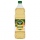We mix 70g water and 50g 8% vinegar. How many percents of the solution of vinegar we produce?